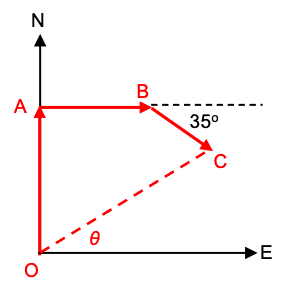# Vector questions with X and Y Components

homeworks
Member warned that homework posts must include an attempt.
Homework Statement:
Vectors
Relevant Equations:
If I walk 20 km North, then 15 km East, then 10 km at 35° South of East

1-What is the magnitude of my displacement?

2-What is the angle of my displacement from east?

3-If I travel the entire distance in 4 hours, then what is my average velocity?
I know what x and y components are but this is really confusing me. And the angle I think is the inverse of tan something. part 3 is so confusing smh

Gold Member
2022 Award
Homework Statement:: Vectors
Relevant Equations:: If I walk 20 km North, then 15 km East, then 10 km at 35° South of East

1-What is the magnitude of my displacement?

2-What is the angle of my displacement from east?

3-If I travel the entire distance in 4 hours, then what is my average velocity?

I know what x and y components are but this is really confusing me. And the angle I think is the inverse of tan something. part 3 is so confusing smh
Draw the path (vector) diagram. That's ALWAYS the way to start these problems.

•Hall
homeworks
Draw the path (vector) diagram. That's ALWAYS the way to start these problems.
I have drawn it already. I did 20km N then 15 km E then 10 km at 35 S of E. I got kind of a rectangle shape but not really. idk what to do next

Staff Emeritus
Homework Helper
Do you know what the words in the question mean? What is displacement? What is average velocity?

•PeroK
homeworks
Do you know what the words in the question mean? What is displacement? What is average velocity?
Yeah displacement is the total distance in a straight line. So I would need to convert 10 km at 35 south of east to x and y component, which I guess would be x-component=10cos35 and y-component=10sin35. then what?

•PeroK
Staff Emeritus
Homework Helper
Yeah displacement is the total distance in a straight line.
Not exactly. For one thing, displacement is a vector whereas distance isn't.

Displacement is the change in position. So the question is asking you how far north and east you end up compared to where you started. Can you figure that out from your diagram?

homeworks
Not exactly. For one thing, displacement is a vector whereas distance isn't.

Displacement is the change in position. So the question is asking you how far north and east you end up compared to where you started. Can you figure that out from your diagram?
No, can you?

•PeroK
Staff Emeritus
Homework Helper
Can you answer the question if it's just the first two legs of the journey?

homeworks
Can you answer the question if it's just the first two legs of the journey?
You mean if it's just 20 km North and 15 km East? Yes. it would be 25

Staff Emeritus
Homework Helper
How far north and east (separately) do you end up compared to where you started?

homeworks
How far north and east (separately) do you end up compared to where you started?
You end up 20 km North and 15 km East from where you started?

Staff Emeritus
Homework Helper
Yup. Note that you treated walking north completely separately from walking east because the two directions are perpendicular to each other.

So now add in the last leg. How far in the northward direction does the last leg take you (negative because you'll be walking southward)? How far east does the last leg take you? Use basic trig to figure those out.

Homework Helper
Gold Member
Here is a drawing drawn to scale that could serve as reference for future discussion (if needed.)
In part (a) you need to find the length of OC
In part (b) you need to find angle θ.
In part (c) you need to apply the definition of average velocity. Look it up if you don't remember it, but keep in mind that it is a vector therefore you have to specify both its magnitude and direction.•jbriggs444, Steve4Physics and Hall
Mentor
I have drawn it already. I did 20km N then 15 km E then 10 km at 35 S of E. I got kind of a rectangle shape but not really. idk what to do next
Not a rectangle, as @kuruman's drawing in the previous post shows.

•